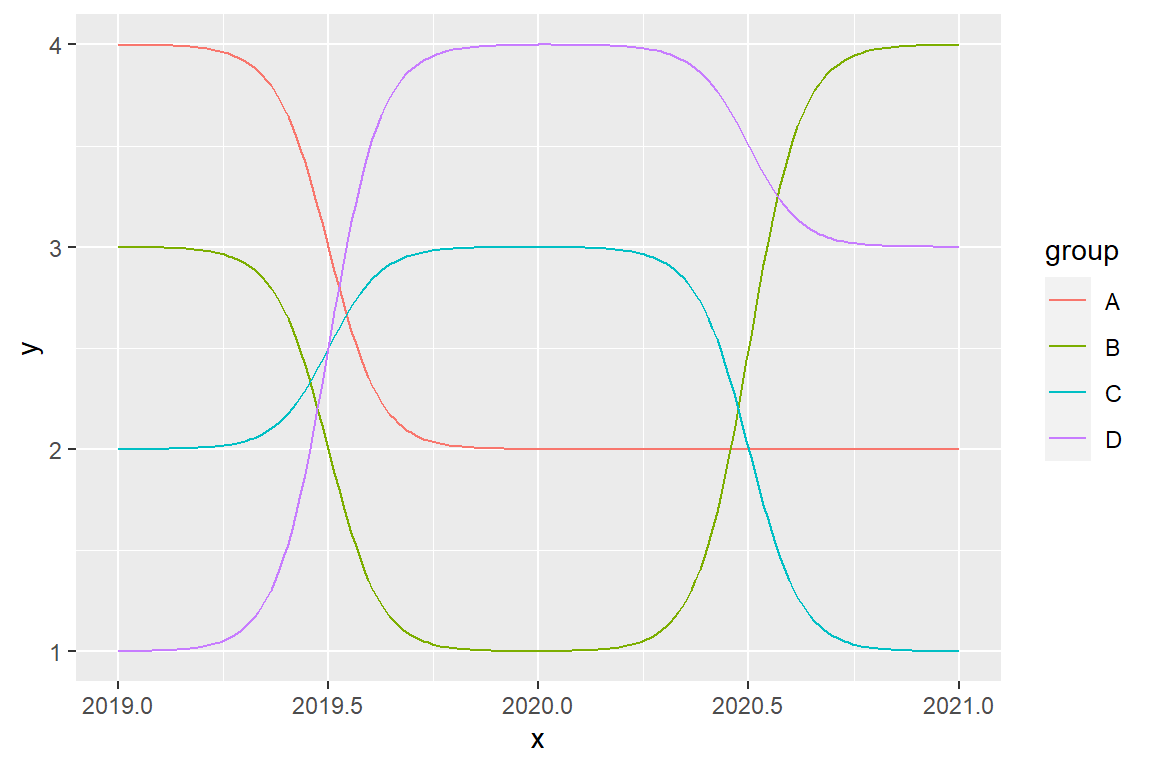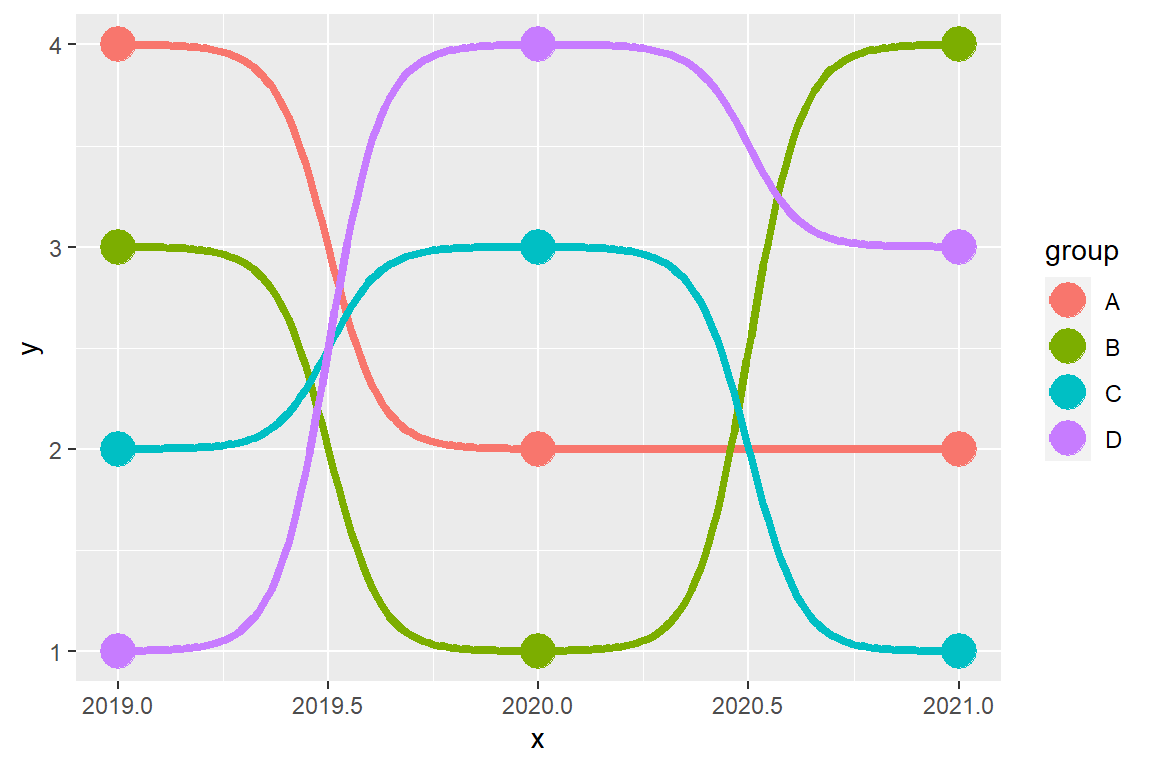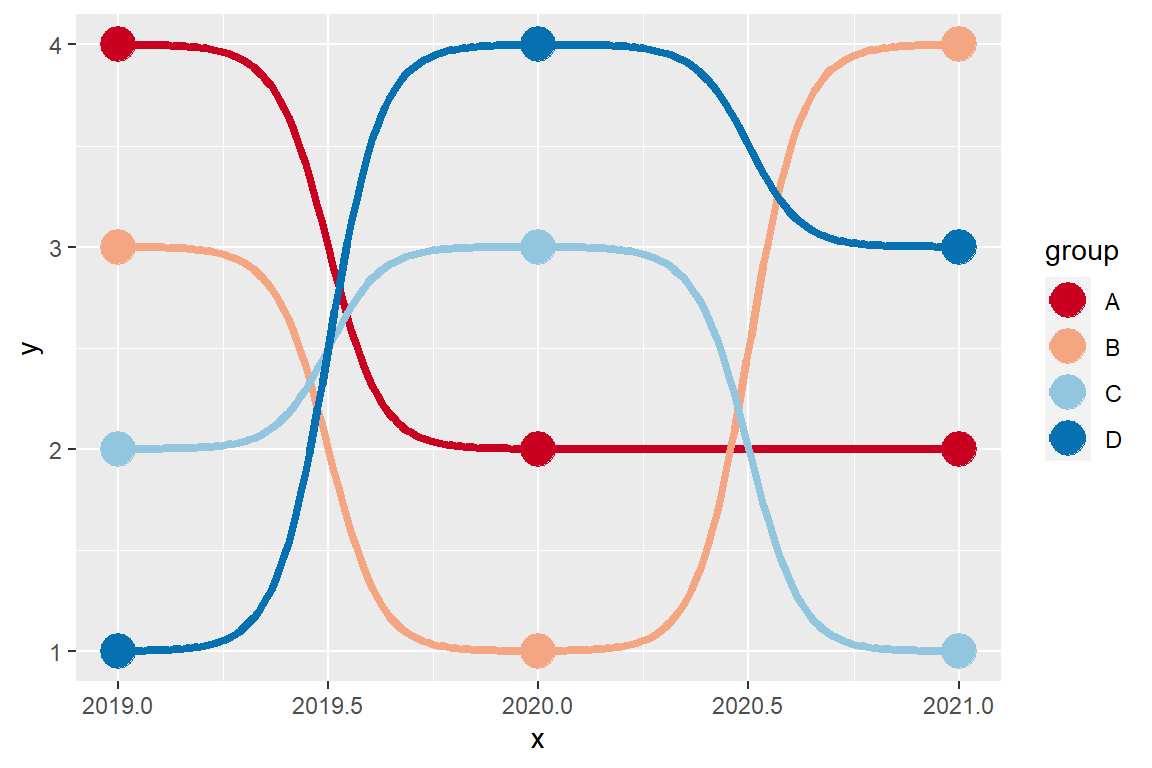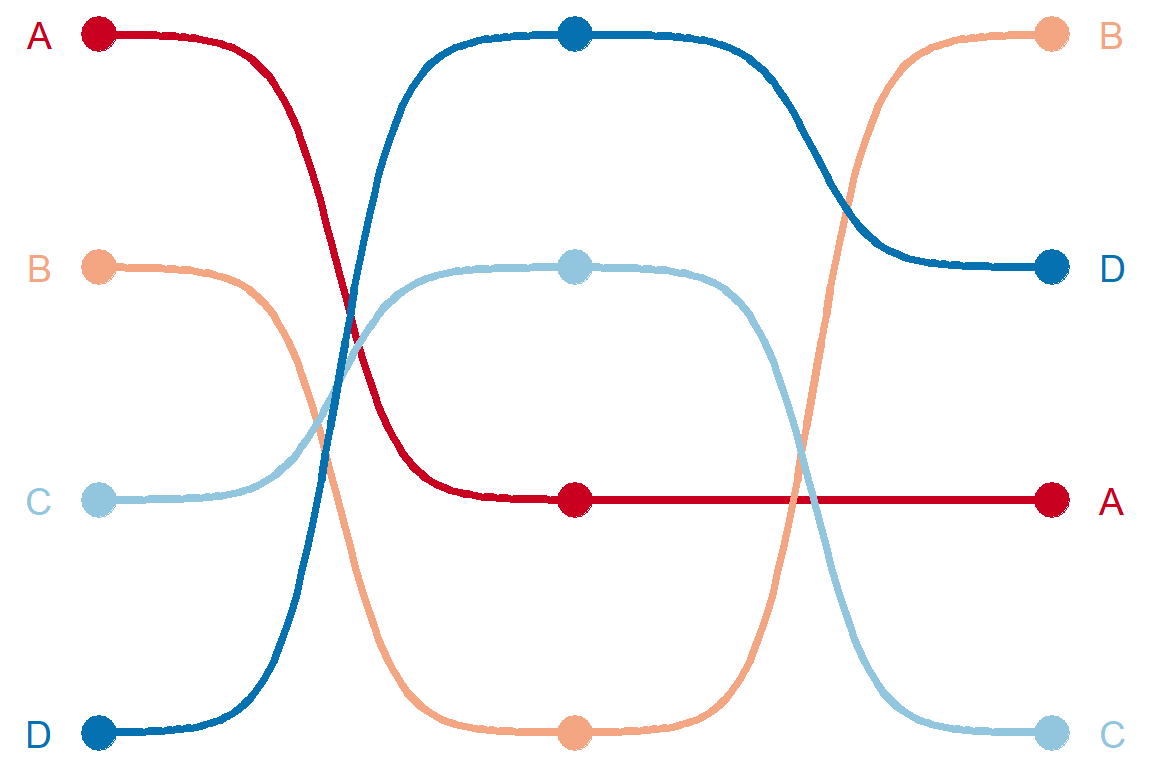# Bump chart in ggplot2 with ggbump

David Sjoberg

## Sample data set

The following data set represents the ranking position (`y`) of some tennis players (`group`) over the years (`x`). This data will be used in the following examples.

``````year <- rep(2019:2021, 4)
position <- c(4, 2, 2, 3, 1, 4, 2, 3, 1, 1, 4, 3)
player <- c("A", "A", "A",
"B", "B", "B",
"C", "C", "C",
"D", "D", "D")

df <- data.frame(x = year,
y = position,
group = player)``````

## Most basic bump chart

In order to create a bump chart in ggplot2 you will need to load the `ggbump` package and the `tidyverse`.

``````# install.packages("tidyverse")
# install.packages("ggbump")
library(tidyverse)
library(ggbump)

ggplot(df, aes(x = x, y = y, color = group)) +
geom_bump()``````## Bump chart customizationYou can add points over the lines with `geom_point`.

``````# install.packages("tidyverse")
# install.packages("ggbump")
library(tidyverse)
library(ggbump)

ggplot(df, aes(x = x, y = y, color = group)) +
geom_bump(size = 1.5) +
geom_point(size = 6)``````

The colors of the lines and points can be changed with a predefined color scale, such as `scale_color_brewer` or setting custom colors with `scale_color_manual`.``````# install.packages("tidyverse")
# install.packages("ggbump")
library(tidyverse)
library(ggbump)

ggplot(df, aes(x = x, y = y, color = group)) +
geom_bump(size = 1.5) +
geom_point(size = 6) +
scale_color_brewer(palette = "RdBu")``````

A further customization can be applied. In the following example we are adding the texts on the vertical sides of the plot to display the change in the rankings.``````# install.packages("tidyverse")
# install.packages("ggbump")
library(tidyverse)
library(ggbump)

ggplot(df, aes(x = x, y = y, color = group)) +
geom_bump(size = 1.5) +
geom_point(size = 6) +
geom_text(data = df %>% filter(x == min(x)),
aes(x = x - 0.1, label = group),
size = 5, hjust = 1) +
geom_text(data = df %>% filter(x == max(x)),
aes(x = x + 0.1, label = group),
size = 5, hjust = 0) +
scale_color_brewer(palette = "RdBu") +
theme_void() +
theme(legend.position = "none")``````